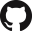# 01.112 Machine Learning¶

No. of Credits: 12 Subject Credits
Pre-requisites:

• A working knowledge of programming in Python or Java and a strong foundation in probability and statistics, and optimization (topics such as dynamic programming)

## Course Description¶

Machine learning is the study of algorithms that improve their performance at some task with experience. In this course, students will learn how machine learning has led to many innovative real-world applications. The students will also gain an in-depth understanding of a broad range of machine learning algorithms from basic to state-of-the-art, such as: naïve Bayes, logistic regression, neural networks, clustering, probabilistic graphical models, reinforcement learning and SVMs.

## Learning Objectives¶

At the end of the term, students will be able to:

1. Recognize the characteristics of machine learning that make it useful to real-world problems.
2. Explain the basic underlying concepts for supervised discriminative and generative learning.
3. Explain the concepts of cross-validation and regularization, be able to use them for estimation of algorithm parameters.
4. Characterize machine learning algorithms as supervised, semi-supervised, and unsupervised.
5. Have heard of a few machine learning toolboxes.
6. Use support vector machines.
7. Use regularized regression algorithms.
8. Explain the concept behind neural networks for learning non-linear functions.
9. Apply unsupervised algorithms for clustering.
10. Explain the foundation of generative models.
11. Implement the inference and learning algorithms for the hidden Markov model.
12. Explain the learning algorithm for hidden Markov model with latent variables.
13. Explain algorithms for learning Bayesian networks.
14. Explain reinforcement learning algorithms.

## Measurable Outcomes¶

1. List useful real-world applications of machine learning.
2. Implement and apply machine learning algorithms.
3. Choose appropriate algorithms for a variety of problems.

## Course Notes¶

This set of course notes was graciously shared by Tey Siew Wen, updated as of 06 February 2020.